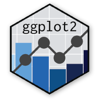Show Sidebar Hide Sidebar# geom_spoke in ggplot2

How to use geom_spoke with Plotly.

### New to Plotly?

Plotly's R library is free and open source!
You can set up Plotly to work in online or offline mode.
We also have a quick-reference cheatsheet (new!) to help you get started!

### Version Check

Version 4 of Plotly's R package is now available!
Check out this post for more information on breaking changes and new features available in this version.

library(plotly)
packageVersion('plotly')

##  '4.9.1'


### Basic Example

library(plotly)

df <- expand.grid(x = 1:10, y=1:10)
df$angle <- runif(100, 0, 2*pi) df$speed <- runif(100, 0, sqrt(0.1 * df\$x))

p <- ggplot(df, aes(x, y)) +
geom_point() +
geom_spoke(aes(angle = angle, radius = speed))

p <- ggplotly(p)

p


Reference: ggplot2 docs

### Reference